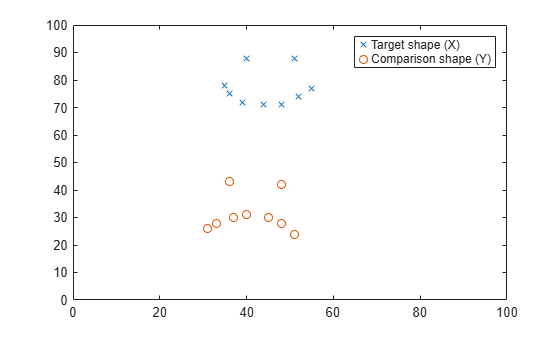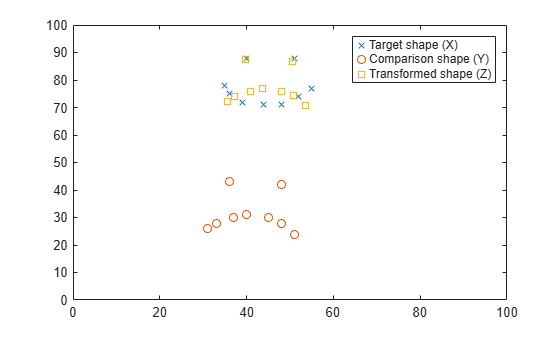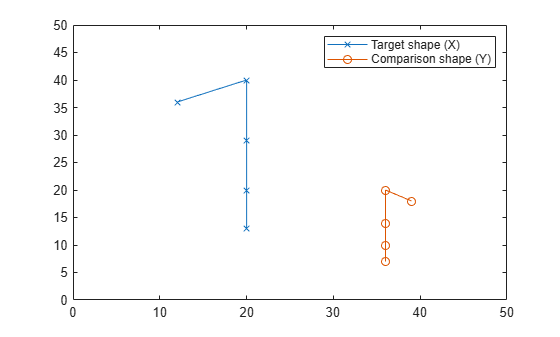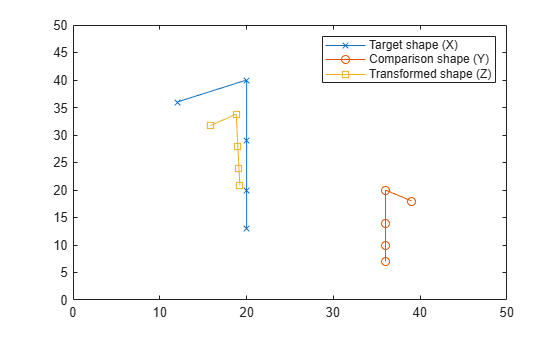# procrustes

Procrustes analysis

## Syntax

``d = procrustes(X,Y)``
``d = procrustes(X,Y,Name,Value)``
``[d,Z] = procrustes(___)``
``[d,Z,transform] = procrustes(___)``

## Description

example

````d = procrustes(X,Y)` returns the Procrustes Distance between the shapes of `X` and `Y`, which are represented by configurations of landmark points.```

example

````d = procrustes(X,Y,Name,Value)` specifies additional options using one or more name-value arguments. For example, you can restrict the Procrustes transformation by disabling reflection and scaling.```
````[d,Z] = procrustes(___)` also returns `Z`, the shape resulting from performing the Procrustes transformation on `Y`, using any of the input argument combinations in the previous syntaxes```

example

````[d,Z,transform] = procrustes(___)` also returns the Procrustes transformation.```

## Examples

collapse all

Construct matrices containing landmark points for two shapes, and visualize the shapes by plotting their landmark points.

```X = [40 88; 51 88; 35 78; 36 75; 39 72; 44 71; 48 71; 52 74; 55 77]; Y = [36 43; 48 42; 31 26; 33 28; 37 30; 40 31; 45 30; 48 28; 51 24]; plot(X(:,1),X(:,2),"x") hold on plot(Y(:,1),Y(:,2),"o") xlim([0 100]) ylim([0 100]) legend("Target shape (X)","Comparison shape (Y)")```Compare the shapes and view their Procrustes distance.

`[d,Z] = procrustes(X,Y)`
```d = 0.2026 ```
```Z = 9×2 39.7694 87.5089 50.5616 86.8011 35.5487 72.1631 37.3131 73.9909 40.8735 75.8503 43.5517 76.7959 48.0577 75.9771 50.7835 74.2286 53.5410 70.6841 ```

Visualize the shape that results from superimposing `Y` onto `X`.

```plot(Z(:,1),Z(:,2),"s") legend("Target shape (X)","Comparison shape (Y)", ... "Transformed shape (Z)") hold off```Use the Procrustes transformation returned by `procrustes` to analyze how it superimposes the comparison shape onto the target shape.

Generate sample data in two dimensions.

```rng("default") n = 10; Y = normrnd(0,1,[n 2]);```

Create the target shape `X` by rotating `Y` 60 degrees (`pi/3` in radians), scaling the size of `Y` by factor 0.5, and then translating the points by adding 2. Also, add some noise to the landmark points in `X`.

`S = [cos(pi/3) -sin(pi/3); sin(pi/3) cos(pi/3)]`
```S = 2×2 0.5000 -0.8660 0.8660 0.5000 ```
`X = normrnd(0.5*Y*S+2,0.05,n,2);`

Find the Procrustes transformation that can transform `Y` to `X`.

`[~,Z,transform] = procrustes(X,Y);`

Display the components of the Procrustes transformation.

`transform`
```transform = struct with fields: T: [2x2 double] b: 0.4845 c: [10x2 double] ```
`transform.T`
```ans = 2×2 0.4832 -0.8755 0.8755 0.4832 ```
`transform.c`
```ans = 10×2 2.0325 1.9836 2.0325 1.9836 2.0325 1.9836 2.0325 1.9836 2.0325 1.9836 2.0325 1.9836 2.0325 1.9836 2.0325 1.9836 2.0325 1.9836 2.0325 1.9836 ```

`transform.T` is similar to the matrix `S`. Also, the scale component (`transform.b`) is close to 0.5, and the translation component values (`transform.c`) are close to 2.

Determine whether `transform.T` indicates a rotation or reflection by computing the determinant of `transform.T`. The determinant of a rotation matrix is `1`, and the determinant of a reflection matrix is –1.

`det(transform.T) `
```ans = 1 ```

In two-dimensional space, a rotation matrix that rotates a point by an angle of $\theta$ degrees about the origin has the form

$\left[\begin{array}{cc}\mathrm{cos}\theta & -\mathrm{sin}\theta \\ \mathrm{sin}\theta & \mathrm{cos}\theta \end{array}\right]$.

If you use either $\mathrm{cos}\theta$ or $\mathrm{sin}\theta$, the rotation angle has two possible values between –180 and 180. Use both the $\mathrm{cos}\theta$ and $\mathrm{sin}\theta$ values to determine the rotation angle of the matrix without ambiguity. Using the `atan2d` function, you can determine the $\mathrm{tan}\theta$ value from $\mathrm{cos}\theta$ and $\mathrm{sin}\theta$, and also determine the angle.

`theta = atan2d(transform.T(2,1),transform.T(1,1))`
```theta = 61.1037 ```

`transform.T` is a rotation matrix of 61 degrees.

Use the Procrustes transformation returned by `procrustes` to analyze how it superimposes the comparison shape onto the target shape.

Create matrices with landmark points for two separate shapes.

```X = [20 13; 20 20; 20, 29; 20 40; 12 36]; Y = [36 7; 36 10; 36 14; 36 20; 39 18];```

Plot the landmark points to visualize the shapes.

```plot(X(:,1),X(:,2),"-x") hold on plot(Y(:,1),Y(:,2),"-o") xlim([0 50]) ylim([0 50]) legend("Target shape (X)","Comparison shape (Y)") hold off```Obtain the Procrustes transformation by using `procrustes`.

`[d,Z,transform] = procrustes(X,Y)`
```d = 0.0064 ```
```Z = 5×2 20.1177 13.3935 19.9145 19.6790 19.6435 28.0597 19.2371 40.6306 13.0871 36.2371 ```
```transform = struct with fields: T: [2x2 double] b: 2.0963 c: [5x2 double] ```
`transform.T`
```ans = 2×2 -0.9995 -0.0323 -0.0323 0.9995 ```
`transform.c`
```ans = 5×2 96.0177 1.1661 96.0177 1.1661 96.0177 1.1661 96.0177 1.1661 96.0177 1.1661 ```

The scale component of the transformation `b` indicates that the scale of `X` is about twice the scale of `Y`.

Find the determinant of the rotation and reflection component of the transformation.

`det(transform.T)`
```ans = -1.0000 ```

The determinant is `–1`, which means that the transformation contains a reflection.

In two-dimensional space, a reflection matrix has the form

$\left[\begin{array}{cc}\mathrm{cos2}\theta & \mathrm{sin2}\theta \\ \mathrm{sin2}\theta & -\mathrm{cos2}\theta \end{array}\right]$,

which indicates a reflection over a line that makes an angle $\theta$ with the x-axis.

If you use either $\mathrm{cos}2\theta$ or $\mathrm{sin}2\theta$, the angle for the line of reflection has two possible values between –90 and 90. Use both the $\mathrm{cos}2\theta$ and $\mathrm{sin}2\theta$ values to determine the angle for the line of reflection without ambiguity. Using the `atan2d` function, you can determine the $\mathrm{tan}2\theta$ value from $\mathrm{cos}2\theta$ and $\mathrm{sin}2\theta$, and also determine the angle.

`theta = atan2d(transform.T(2,1),transform.T(1,1))/2`
```theta = -89.0741 ```

`transform.T` reflects points across a line that makes roughly a –90 degree angle with the x-axis; this line indicates the y-axis. The plots of `X` and `Y` show that reflecting across the y-axis is required to superimpose `Y` onto `X`.

Find the Procrustes transformation for landmark points, and apply the transformation to more points on the comparison shape than just the landmark points.

Create matrices with landmark points for two triangles `X` (target shape) and `Y` (comparison shape).

```X = [5 0; 5 5; 8 5]; Y = [0 0; 1 0; 1 1];```

Create a matrix with more points on the triangle `Y`.

```Y_points = [linspace(Y(1,1),Y(2,1),10)' linspace(Y(1,2),Y(2,2),10)' linspace(Y(2,1),Y(3,1),10)' linspace(Y(2,2),Y(3,2),10)' linspace(Y(3,1),Y(1,1),10)' linspace(Y(3,2),Y(1,2),10)'];```

Plot both shapes, including the larger set of points for the comparison shape.

```plot([X(:,1); X(1,1)],[X(:,2); X(1,2)],"bx-") hold on plot([Y(:,1); Y(1,1)],[Y(:,2); Y(1,2)],"ro-","MarkerFaceColor","r") plot(Y_points(:,1),Y_points(:,2),"ro") xlim([-1 10]) ylim([-1 6]) legend("Target shape (X)","Comparison shape (Y)", ... "Additional points on Y","Location","northwest")```Call `procrustes` to obtain the Procrustes transformation from the comparison shape to the target shape.

`[d,Z,transform] = procrustes(X,Y)`
```d = 0.0441 ```
```Z = 3×2 5.0000 0.5000 4.5000 4.5000 8.5000 5.0000 ```
```transform = struct with fields: T: [2x2 double] b: 4.0311 c: [3x2 double] ```

Use the Procrustes transformation to superimpose the other points (`Y_points`) on the comparison shape onto the target shape, and then visualize the results.

```Z_points = transform.b*Y_points*transform.T + transform.c(1,:); plot([Z(:,1); Z(1,1)],[Z(:,2); Z(1,2)],"ks-","MarkerFaceColor","k") plot(Z_points(:,1),Z_points(:,2),"ks") legend("Target shape (X)","Comparison shape (Y)", ... "Additional points on Y","Transformed shape (Z)", ... "Transformed additional points","Location","best") hold off```Construct the shapes of the handwritten letters d and b using landmark points, and then plot the points to visualize the letters.

```D = [33 93; 33 87; 33 80; 31 72; 32 65; 32 58; 30 72; 28 72; 25 69; 22 64; 23 59; 26 57; 30 57]; B = [48 83; 48 77; 48 70; 48 65; 49 59; 49 56; 50 66; 52 66; 56 65; 58 61; 57 57; 54 56; 51 55]; plot(D(:,1),D(:,2),"x-") hold on plot(B(:,1),B(:,2),"o-") legend("Target shape (d)","Comparison shape (b)") hold off```Use `procrustes` to compare the letters with reflection turned off, because reflection would turn the b into a d and not accurately preserve the shape you want to compare.

`d = procrustes(D,B,"reflection",false)`
```d = 0.3425 ```

Try using `procrustes` with reflection on to see how the Procrustes distance differs.

`d = procrustes(D,B,"reflection","best")`
```d = 0.0204 ```

This reflection setting results in a smaller Procrustes distance because reflecting b better aligns it with d.

Construct two shapes represented by their landmark points, and then plot the points to visualize them.

```X = [20 13; 20 20; 20 29; 20 40; 12 36]; Y = [36 7; 36 10; 36 14; 36 20; 39 18]; plot(X(:,1),X(:,2),"-x") hold on plot(Y(:,1),Y(:,2),"-o") xlim([0 50]) ylim([0 50]) legend("Target shape (X)","Comparison shape (Y)")```Compare the two shapes using Procrustes analysis with scaling turned off.

`[d,Z] = procrustes(X,Y,"scaling",false)`
```d = 0.2781 ```
```Z = 5×2 19.2194 20.8229 19.1225 23.8214 18.9932 27.8193 18.7993 33.8162 15.8655 31.7202 ```

Visualize the superimposed landmark points.

```plot(Z(:,1),Z(:,2),"-s") legend("Target shape (X)","Comparison shape (Y)", ... "Transformed shape (Z)") hold off```The superimposed shape `Z` does not differ in scale from the original shape `Y`.

## Input Arguments

collapse all

Target shape, specified as an n-by-p matrix where each of the n rows contains a p-dimensional landmark point. The landmark points represent the shape that is the target of the comparison.

Data Types: `single` | `double`

Comparison shape, specified as an n-by-q matrix where each of the n rows contains a q-dimensional landmark point with qp. The landmark points represent the shape to be compared with the target shape.

`Y` must have the same number of points (rows) as `X`, where each point in `Y`, `Y(i,:)` corresponds to the point in the same row in `X`, `X(i,:)`.

Points in `Y` can have fewer dimensions (number of columns) than points in `X`. In this case, `procrustes` appends columns of zeros to `Y` to match the dimensions of `X`.

Data Types: `single` | `double`

### Name-Value Arguments

Specify optional pairs of arguments as `Name1=Value1,...,NameN=ValueN`, where `Name` is the argument name and `Value` is the corresponding value. Name-value arguments must appear after other arguments, but the order of the pairs does not matter.

Before R2021a, use commas to separate each name and value, and enclose `Name` in quotes.

Example: `d = procrustes(X,Y,"Scaling",false,"reflection",false)` performs Procrustes analysis without scaling or reflection in the transformation.

Flag to enable scaling in the Procrustes transformation, specified as logical `1` (`true`) or `0` (`false`). A value of `false` prevents scaling in the transformation. A value of `true` allows scaling if it minimizes the differences between the landmark points in `X` and `Y`.

Set `Scaling` to `false` to compare `Y` to `X` without scaling `Y` to match the scale of `X`. This option causes shapes of different scales to have a greater Procrustes distance.

Example: `"Scaling",false`

Data Types: `logical`

Flag to enable reflection in the Procrustes transformation, specified as `"best"`, logical `1` (`true`), or logical `0` (`false`).

• `"best"` — Find the optimal Procrustes transformation, regardless of whether or not it contains a reflection.

• `1` (`true`) — Force the Procrustes transformation to reflect `Y`, whether or not the transformation minimizes the differences between the landmark points.

• `0` (`false`) — Prevent the Procrustes transformation from reflecting `Y`. This option does not prevent rotation in the transformation.

Set `Reflection` to `false` to compare `Y` to `X` without reflecting `Y` to match the shape of `X`. This option causes shapes that are reflections of each other to have a greater Procrustes distance.

Example: `"Reflection",true`

Data Types: `logical` | `string` | `char`

## Output Arguments

collapse all

Procrustes distance, a measure of dissimilarity between two shapes, returned as a numeric scalar in the range `[0,1]`. If `Scaling` is set to `false`, the Procrustes distance can be outside of the range `[0,1]`.

`procrustes` computes the distance using the sum of squared differences between the corresponding points in `X` and `Z`. The function then standardizes the Procrustes distance by the scale of `X`. The scale of `X` is `sum(sum((X-mean(X)).^2))`, which is the sum of squared elements of a centered version of `X` where the columns of `X` have mean `0`.

Data Types: `single` | `double`

Transformed shape of the landmark points in `Y`, returned as an n-by-p numeric matrix that is the same size as `X`. The output `Z` is the result of applying the Procrustes transformation to `Y`.

Data Types: `single` | `double`

Procrustes transformation, returned as a structure with three fields:

• `T` — Rotation and reflection component, specified by a p-by-p transformation matrix that rotates or reflects `Y` to match the orientation of the landmark points in `X`.

• If `T` is a rotation matrix, then `det(T)` is 1.

• If `T` is a reflection matrix, then `det(T)` is –1.

• `b` — Scale component, specified by a scalar to stretch (`b` > 1), conserve (`b` = 1), or shrink (`b` < 1) the scale of `Y` to match the scale of `X`.

• `c` — Translation component, specified by an n-by-p matrix where each row is the p-dimensional vector to add to the points in `Y` to shift it onto `X`.

The Procrustes transformation superimposes `Y` onto `X` by performing the following transformation:

Z = bYT + c.

Set the `Reflection` name-value argument to `false` to ensure that `transform.T` does not contain a reflection.

Set the `Scaling` name-value argument to `false` to remove the scale component, fixing `transform.b` to `1`.

Data Types: `struct`

collapse all

### Procrustes Distance

The Procrustes distance is a measure of dissimilarity between shapes based on Procrustes analysis.

The `procrustes` function finds the Procrustes transformation, which is the best shape-preserving Euclidean transformation (consisting of rotation, reflection, scaling, and translation) between the two shapes `X` and `Y`. The Procrustes transformation is an optimal transformation that minimizes the sum of squared differences between the landmark points in `X` and `Z`, where `Z` is the transformed shape of `Y` that results from superimposing `Y` onto `X`.

The `procrustes` function returns the Procrustes distance (`d`), transformed shape (`Z`), and Procrustes transformation (`transform`). The Procrustes distance is the sum of squared differences between `X` and `Z`.

## Tips

• Procrustes analysis is appropriate when all dimensions in `X` and `Y` have similar scales. If the columns of `X` and `Y` have different scales, standardize the columns by using `zscore` or `normalize`.

• Procrustes analysis is useful in conjunction with multidimensional scaling. Two different applications of multidimensional scaling can produce reconstructed points that are similar in principle, but look different because they have different orientations. Also, the reconstructed points can have a different orientation than the original points. The `procrustes` function transforms one set of points to make them more comparable to the other. For an example, see Classical Multidimensional Scaling Applied to Nonspatial Distances.

 Kendall, David G. “A Survey of the Statistical Theory of Shape.” Statistical Science. Vol. 4, No. 2, 1989, pp. 87–99.

 Bookstein, Fred L. Morphometric Tools for Landmark Data. Cambridge, UK: Cambridge University Press, 1991.

 Seber, G. A. F. Multivariate Observations. Hoboken, NJ: John Wiley & Sons, Inc., 1984.